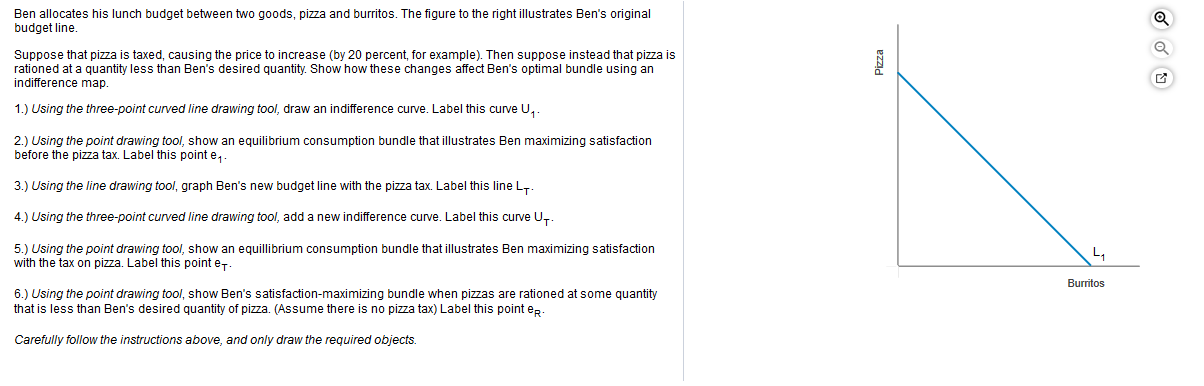# Ben allocates his lunch budget between two goods, pizza and burritos. The figure to the right illustrates Ben's originalbudget line.Suppose that pizza is taxed, causing the price to increase (by 20 percent, for example). Then suppose instead that pizza isrationed at a quantity less than Ben's desired quantity. Show how these changes affect Ben's optimal bundle using anindifference map.1.) Using the three-point curved line drawing tool, draw an indifference curve. Label this curve U2.) Using the point drawing tool, show an equilibrium consumption bundle that illustrates Ben maximizing satisfactionbefore the pizza tax. Label this point e3.) Using the line drawing tool, graph Ben's new budget line with the pizza tax. Label this line LT4.) Using the three-point curved line drawing tool, add a new indifference curve. Label this curve U5.) Using the point drawing tool, show an equillibrium consumption bundle that illustrates Ben maximizing satisfactionwith the tax on pizza. Label this point eL.Burritos6.) Using the point drawing tool, show Ben's satisfaction-maximizing bundle when pizzas are rationed at some quantitythat is less than Ben's desired quantity of pizza. (Assume there is no pizza tax) Label this point epCarefully follow the instructions above, and only draw the required objectsPizza

Question
529 viewshelp_outlineImage TranscriptioncloseBen allocates his lunch budget between two goods, pizza and burritos. The figure to the right illustrates Ben's original budget line. Suppose that pizza is taxed, causing the price to increase (by 20 percent, for example). Then suppose instead that pizza is rationed at a quantity less than Ben's desired quantity. Show how these changes affect Ben's optimal bundle using an indifference map. 1.) Using the three-point curved line drawing tool, draw an indifference curve. Label this curve U 2.) Using the point drawing tool, show an equilibrium consumption bundle that illustrates Ben maximizing satisfaction before the pizza tax. Label this point e 3.) Using the line drawing tool, graph Ben's new budget line with the pizza tax. Label this line LT 4.) Using the three-point curved line drawing tool, add a new indifference curve. Label this curve U 5.) Using the point drawing tool, show an equillibrium consumption bundle that illustrates Ben maximizing satisfaction with the tax on pizza. Label this point e L. Burritos 6.) Using the point drawing tool, show Ben's satisfaction-maximizing bundle when pizzas are rationed at some quantity that is less than Ben's desired quantity of pizza. (Assume there is no pizza tax) Label this point ep Carefully follow the instructions above, and only draw the required objects Pizza fullscreen
check_circle

Step 1

1.

The initial budget line of the consumer is given and the indifference curve of the individual can be drawn as follows:

Step 2

2.

The equilibrium point that indicates that the consumer maximizes the utility is indicated at the point where the indifference curve of the individual is tangent to the budget line of the individual. It is indicated at the point e1 on the above graph.

Step 3

3.

When the pizza is taxed, the price of the pizza would increase in the market. This means that the maximum quantity of pizza that can be consumed by the consumer decreases. Since ...

### Want to see the full answer?

See Solution

#### Want to see this answer and more?

Solutions are written by subject experts who are available 24/7. Questions are typically answered within 1 hour.*

See Solution
*Response times may vary by subject and question.
Tagged in